# vs.eyeandcontacts.com

## Chapter 14  Symmetry Exercise 14.2

Question 1: Which of the following figures have rational symmetry of order more than 1: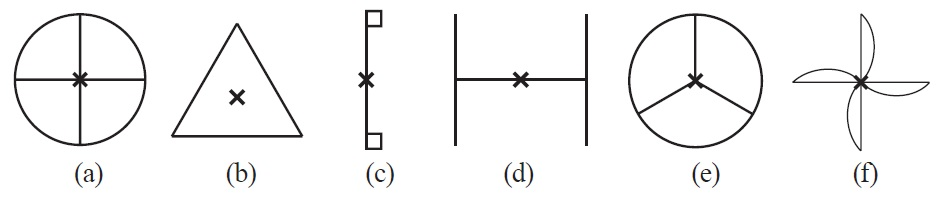(a)The above figure has its rotational symmetry as 4.

(b)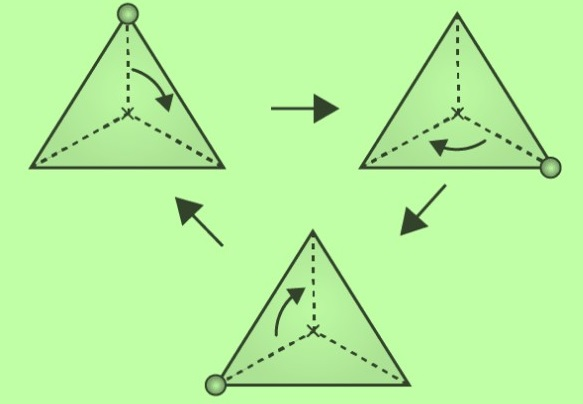The above figure has its rotational symmetry as 3.

(c) The given figure has only one rotational symmetry.

(d)The above figure has its rotational symmetry as 2.

(e)

The above figure has its rotational symmetry as 3.

(f)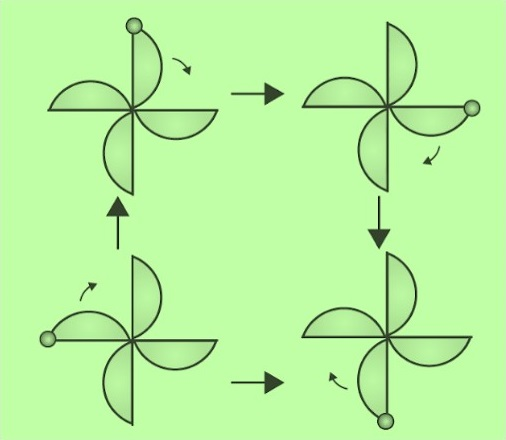So, the above figure has its rotational symmetry as 4.

Therefore, figures (a), (b), (c), (d), (e) and (f) have rotational symmetry of order more than 1.

Question 2: Give the order of rotational symmetry for each figure:
(a)Answer: The above figure has its rotational symmetry as 2.

(b)The above figure has its rotational symmetry as 2.

(c)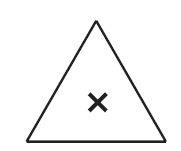The above figure has its rotational symmetry as 3.

(d)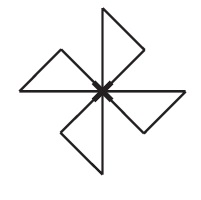The above figure has its rotational symmetry as 4.

(e)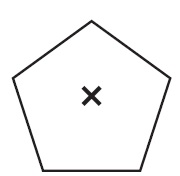The above figure has its rotational symmetry as 4.

(f)The above figure has its rotational symmetry as 5.

(g)